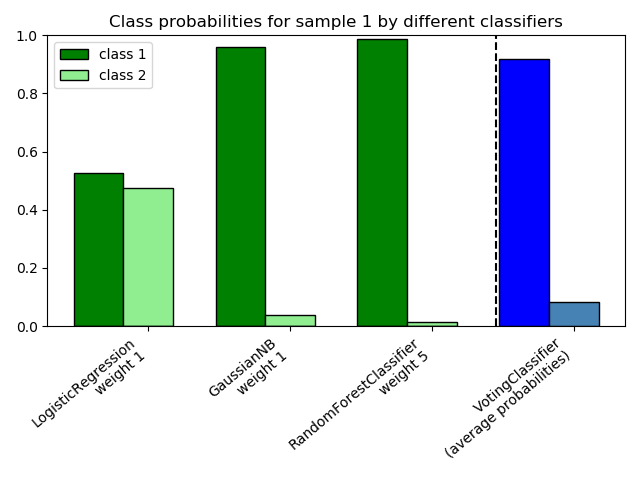# Plot class probabilities calculated by the VotingClassifier¶

Plot the class probabilities of the first sample in a toy dataset predicted by three different classifiers and averaged by the VotingClassifier.

First, three examplary classifiers are initialized (LogisticRegression, GaussianNB, and RandomForestClassifier) and used to initialize a soft-voting VotingClassifier with weights [1, 1, 5], which means that the predicted probabilities of the RandomForestClassifier count 5 times as much as the weights of the other classifiers when the averaged probability is calculated.

To visualize the probability weighting, we fit each classifier on the training set and plot the predicted class probabilities for the first sample in this example dataset.print(__doc__)

import numpy as np
import matplotlib.pyplot as plt

from sklearn.linear_model import LogisticRegression
from sklearn.naive_bayes import GaussianNB
from sklearn.ensemble import RandomForestClassifier
from sklearn.ensemble import VotingClassifier

clf1 = LogisticRegression(solver='lbfgs', max_iter=1000, random_state=123)
clf2 = RandomForestClassifier(n_estimators=100, random_state=123)
clf3 = GaussianNB()
X = np.array([[-1.0, -1.0], [-1.2, -1.4], [-3.4, -2.2], [1.1, 1.2]])
y = np.array([1, 1, 2, 2])

eclf = VotingClassifier(estimators=[('lr', clf1), ('rf', clf2), ('gnb', clf3)],
voting='soft',
weights=[1, 1, 5])

# predict class probabilities for all classifiers
probas = [c.fit(X, y).predict_proba(X) for c in (clf1, clf2, clf3, eclf)]

# get class probabilities for the first sample in the dataset
class1_1 = [pr[0, 0] for pr in probas]
class2_1 = [pr[0, 1] for pr in probas]

# plotting

N = 4  # number of groups
ind = np.arange(N)  # group positions
width = 0.35  # bar width

fig, ax = plt.subplots()

# bars for classifier 1-3
p1 = ax.bar(ind, np.hstack(([class1_1[:-1], ])), width,
color='green', edgecolor='k')
p2 = ax.bar(ind + width, np.hstack(([class2_1[:-1], ])), width,
color='lightgreen', edgecolor='k')

# bars for VotingClassifier
p3 = ax.bar(ind, [0, 0, 0, class1_1[-1]], width,
color='blue', edgecolor='k')
p4 = ax.bar(ind + width, [0, 0, 0, class2_1[-1]], width,
color='steelblue', edgecolor='k')

# plot annotations
plt.axvline(2.8, color='k', linestyle='dashed')
ax.set_xticks(ind + width)
ax.set_xticklabels(['LogisticRegression\nweight 1',
'GaussianNB\nweight 1',
'RandomForestClassifier\nweight 5',
'VotingClassifier\n(average probabilities)'],
rotation=40,
ha='right')
plt.ylim([0, 1])
plt.title('Class probabilities for sample 1 by different classifiers')
plt.legend([p1, p2], ['class 1', 'class 2'], loc='upper left')
plt.tight_layout()
plt.show()


Total running time of the script: ( 0 minutes 0.245 seconds)

Gallery generated by Sphinx-Gallery